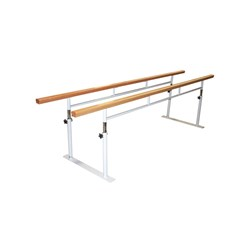# Balance & Stability

Grid List
\$34.00
Increase value Decrease value
\$61.86
Increase value Decrease value3 Meter Folding Walking Rails
\$1418.04
Increase value Decrease value
\$50.00
Increase value Decrease value
\$299.00
Increase value Decrease value
\$340.00
Increase value Decrease value
Alpha Sport Balance Beam Long (195cm)
\$218.90
Increase value Decrease value
\$2.10
Increase value Decrease value
\$44.80
Increase value Decrease value
\$59.10
Increase value Decrease value
\$62.71
Increase value Decrease value
\$66.50
Increase value Decrease value
\$199.00
Increase value Decrease value
\$65.23
Increase value Decrease value
\$220.00
Increase value Decrease value
\$199.00
Increase value Decrease value
\$24.99
Increase value Decrease value
\$50.00
Increase value Decrease value
\$44.99
Increase value Decrease value
\$34.95
Increase value Decrease value
From
Increase value Decrease value
From
Increase value Decrease value
From
Increase value Decrease value
From
Increase value Decrease value
Grid List
##### Products to compare:
Comparing Products